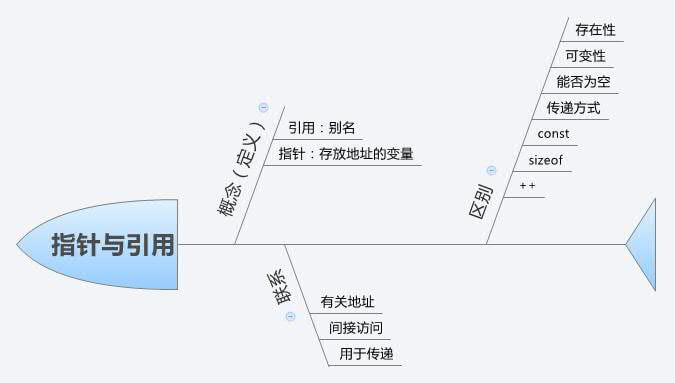## 概念

### 引用

``````int m;
int &n = m;``````

### 指针

（这三句摘自《C++ Primer, Fourth Edition》）。再举个例子：

``````int m;
int *n = &m;``````

## 联系

• 都是有关地址的概念。 指针指向一块内存，它的内容是所指内存的地址；而引用则是某块内存的别名。
• 都是间接访问其他对象。
• 都能用于函数参数和返回值的传递。

## 区别

• 一、指针是一个实体（本质上就是存放变量地址的一个变量），而引用只是个别名。

• 二、指针可变，引用不可变。

• 三、指针可以为空，引用不能为空。

• 四、传递方式不同。

1. 值传递
2. 指针传递
3. 引用传递

``````#include <iostream>
using namespace std;

void f(int *n)
{
*n = 5;
}

int main()
{
int m = 1;
cout << m << endl;
f(&m);
cout << m << endl;

return 0;
}``````

``````#include <iostream>
using namespace std;

void f(int &n)
{
n = 5;
}

int main()
{
int m = 1;
cout << m << endl;
f(m);
cout << m << endl;

return 0;
}``````

`n``f`中的局部变量，同时`n`也是实参`m`的引用。此时`n`就为`m`，所以在`f`中对`n`的操作都会影响`m`，为`n`赋值 5 就是对`m`赋值 5。

• 五、引用不能 const，指针能 const，const 的指针不可变。 对于引用，具体指没有 int&const a 这种形式，而 const int& a 是有的， 前者指引用本身（即别名）不可以改变，这是理所当然的，所以不需要这种形式，后者指引用所指的值不可以改变）

• 六、「sizeof 引用」得到的是所指向的变量(对象)的大小，而“sizeof 指针”得到的是指针本身的大小。

• 七、指针和引用的自增(++)运算意义不一样（很好理解）。